# Scientific Calculator | Complex Number Calculator

Easy-to-use Scientific Calculator with a unique user interface and useful features. Scientific Calculator with Complex Numbers, Statistics and Linear Regression and Graphics. Easy-to-use Scientific Calculator with a unique user interface and useful features.

• Calculate expressions containing complex numbers in standard form a+bi and polar (phasor) form r∠(θ).
• Supports all standard mathematical functions plus the Gamma, Γ, and Psi, Ψ, functions.

Swipe the number pad to the right to see more functions (trig, hyperbolic, inverses, etc).
• Fixed, scientific and engineering notations.

Do statistics with one or two dimensional data:
• x1, x2 ,x3, ….
• Press Σ to calculate sum, mean, max, min, variance, sample and standard deviation, median,upper and lower quartile, etc.

• x1, y1; x2, y2; x3, y3; … .
• Press Σ to calculate the equation of the linear regression line and graph it .
• Long press ! to calculate combinations C(n, r) and permutations P(n, r).
• Enter functions f(x) or parametric equations p(t) and generate table of values.
• Physical/Chemical/Atomic and other scientific constants.
• Easy to use unit converter. (Time, Mass, Length, Velocity, and many more.)

All of the above with more details are included in the built-in Instruction menu accompanying this scientific calculator.

What’s New
Bug fixes.

Screenshots

•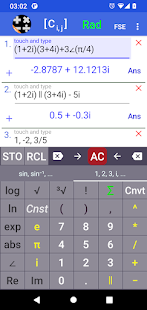•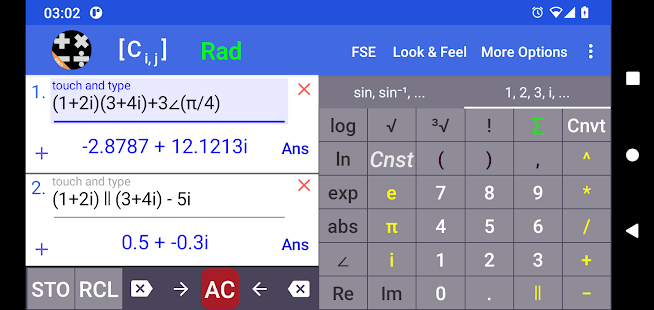•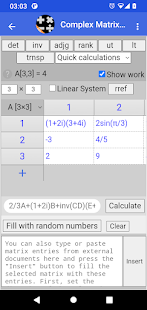•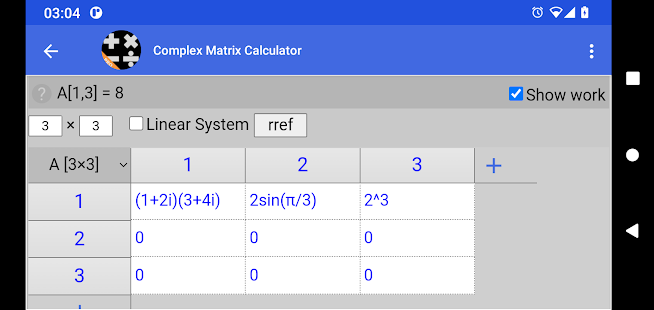••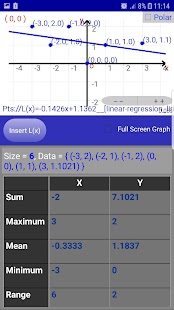•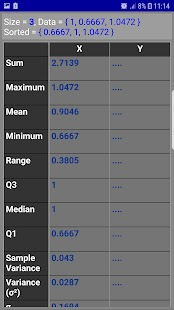••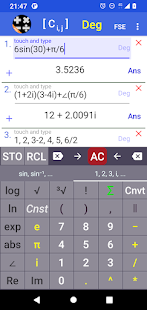•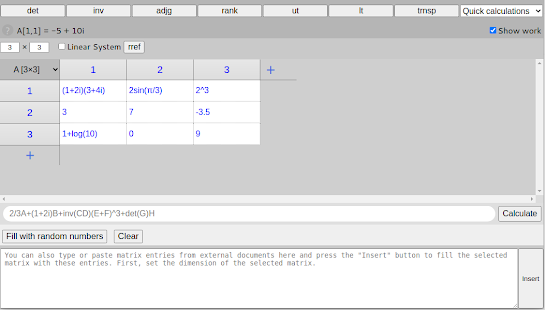••••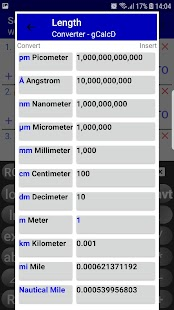•••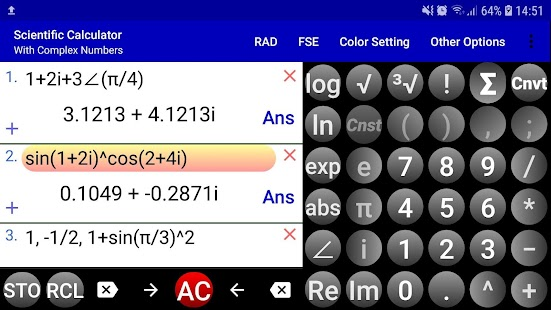•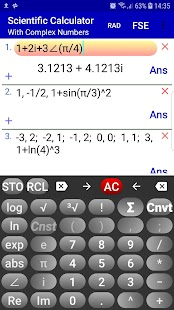•